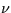Next: 12.5 Vector Displacement FITS Up: 12 Final Archive Data Previous: 12.3 Raw Image FITS

# 12.4 Linearized Image FITS File (LILO/LIHI)

The LILO/LIHI contains linearized (i.e., photometrically-corrected) pixels expressed in flux number (FN) units and situated in RI space. Only the pixels in a swath along the spectrum (low dispersion) and inside the target ring (high dispersion) have been photometrically corrected. The actual FN values have been scaled up by a factor of 32 for storage. The LILO/LIHI contains the LI as a 2-D primary array consisting of 768 × 768 pixels, with each pixel value coded as 16-bit, two's complement integers with bits stored in decreasing order of significance.

The associatedflags are stored as a 2-D array the same size as the LI data, in a FITS image extension using 16-bit, two's complement integers. No scaling is used for the array offlags. For every pixel that is photometrically corrected, this image contains a correspondingflag describing specific error conditions (if applicable) in the LI. Flagged pixels include those which suffer from saturation, are close to the edge of the photometric correction region, or require ITF curve extrapolation to compute an FN value. In addition, all pixels that have not been photometrically corrected, or are known to suffer from bright spots, reseaux, microphonics and/or missing minor frames, are appropriately flagged. Checking for microphonic noise is performed over the entire 768 × 768 image for the LWR camera only. Each error condition is flagged by setting specific bits in the data quality integer array. (See Chapter 3 for more information on how the various error conditions are encoded.) Basic keywords in the main header and the image extension header are shown in Table 12.6.

Table 12.6:  LILO/LIHI - Basic FITS Keywords
Keyword and value Description
 SIMPLE = T Standard FITS Format
 BITPIX = 16 16-bit 2's complement pixels
 NAXIS = 2 Two-dimensional image
 NAXIS1 = 768 Dimension along x-axis
 NAXIS2 = 768 Dimension along y-axis
 EXTEND = T Extensions are present
 CTYPE1 = 'SAMPLE '  x-axis
 CTYPE2 = 'LINE '  y-axis
 BUNIT = 'FN '  Flux Numbers
 BSCALE = 3.1250E-02 real=tape*bscale+bzero
 BZERO = 0. offset
 TELESCOP= 'IUE '  International Ultraviolet Explorer
 FILENAME= 'AAAnnnnn.LIdd'  Filename(camera)(number).LI(disp)
 DATE = 'dd/mm/yy'  Date file was written
 ORIGIN = 'VILSPA '  Institution generating the file
 DATAMIN = nnnnn.n  Minimum pixel value
 DATAMAX = nnnnn.n  Maximum pixel value
 XTENSION= 'IMAGE '  Image extension
 BITPIX = 16 16-bit, 2's complement pixels
 NAXIS = 2 Two_dimensional image
 NAXIS1 = 768 Dimension along the x-axis
 NAXIS2 = 768 Dimension along the y-axis
 PCOUNT = 0 Number of bytes following data matrix
 GCOUNT = 1 Number of groups
 CTYPE1 = 'SAMPLE '  x-axis
 CTYPE2 = 'LINE '  y-axis
 BUNIT = ' '  Unitless
 FILENAME= 'AAAnnnnn.LFdd'  Filename (camera)(number).LF(disp)
 EXTNAME = 'LIF '  LIF pixel quality flagsNext: 12.5 Vector Displacement FITS Up: 12 Final Archive Data Previous: 12.3 Raw Image FITS
Karen Levay
12/4/1997